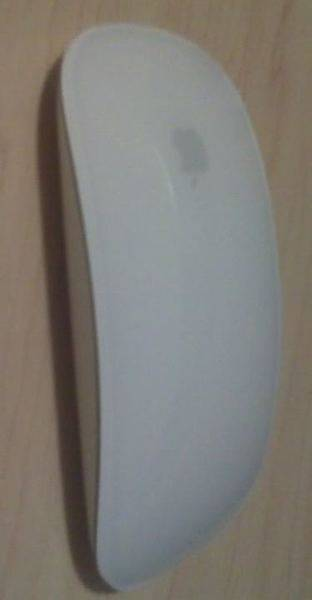2 Optimization ProblemsHeres what i did for this one.
find an equation relating work + math = 16 (total hours she has in a day).
so math = 16 - work (i plug that into the equation for h)
and for money, i have y = 6*work + 6
i plug this in as well and take the derivative.
i get f ' = -4/3 *(6worrk + 6)^-2/3 (16-work)^-1/3

critical points work = -1, 16. the answer is it hits a max at 14/3 hours. what am i doing wrong?i really just need some hints, i have no idea how to get started.

thanks!

jamesrc
Gold Member
For the first one, you need to use the product rule to find the derivative:
if f(x) = u(x)*v(x), then f' = u(dv/dx) + v(du/dx)
then solve for critical points. Critical points occur when the derivative = 0. In your work above, you solved for where the denominator = 0 (making the derivative undefined), so be careful with that when you attempt the problem again.
For the second one, you need to write a function for the total length of the trip to get started. If you travel in the manner described from the origin to a point (x,y), then you first move x to the right, y up, x to the left, then y back down to (0,0). So your total trip is d = 2x+2y. Use the curve given to write d as a function of a single variable, then optimize.

i'm working on the first, after setting the derivative equal to zero, i get

6 h^2/3 / y^2/3 = 2 y^1/3 / h^1/3
before substituing in h = 16 - work and y = 6*work +6
how do i solve this equation?

jamesrc
Gold Member
Your equation is correct so far, but I think you're making things tougher on yourself than they have to be. I would rewrite U as a function of w (my variable for the number of hours worked) and only w. Then you can take the derivative and find the optimum w.

Anyway, the way to solve your equation is to cross multiply, so you get that 2y=6h (which can be solved for w).

jamesrc said:
For the second one, you need to write a function for the total length of the trip to get started. If you travel in the manner described from the origin to a point (x,y), then you first move x to the right, y up, x to the left, then y back down to (0,0). So your total trip is d = 2x+2y. Use the curve given to write d as a function of a single variable, then optimize.

there are 3 variables, d x and y, i'm not sure how to relate them into one. also, how do the equations relate?
and thanks for the help, i got the other question!

jamesrc
Gold Member
No problem.

You've got d(x,y)=2x+2y, which is what you are trying to maximize.
You've also got y = 4-x2/9
Substitute that into the first one so you have d=d(x) (d as a function of x).
Then find the derivative of d with respect to x.
When you set that equal to zero, you will be able to find your critical points and then find where d is a maximum.

jamesrc said:
No problem.

You've got d(x,y)=2x+2y, which is what you are trying to maximize.
You've also got y = 4-x2/9
Substitute that into the first one so you have d=d(x) (d as a function of x).
Then find the derivative of d with respect to x.
When you set that equal to zero, you will be able to find your critical points and then find where d is a maximum.

just got it,
thanks again for all this help!Last edited: